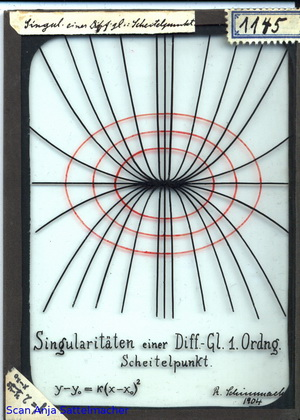Göttingen Collection of Mathematical Models and Instruments

# Slide: Singularities of a differential equation of 1. order

### Model 1145Category: J 43

### Description

Slide: Singularities of a differential equation of 1. order: angular point y-y0=K(x-x0)2